## SUPER COMPUTERS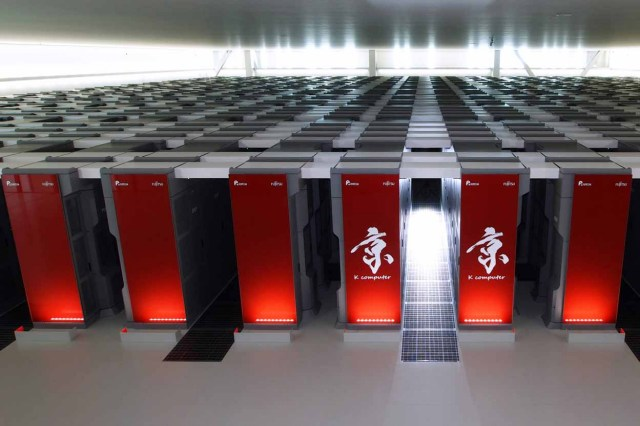## CRAY SUPERCOMPUTERS THE FASTEST COMPUTERS IN THE WORLD in 1982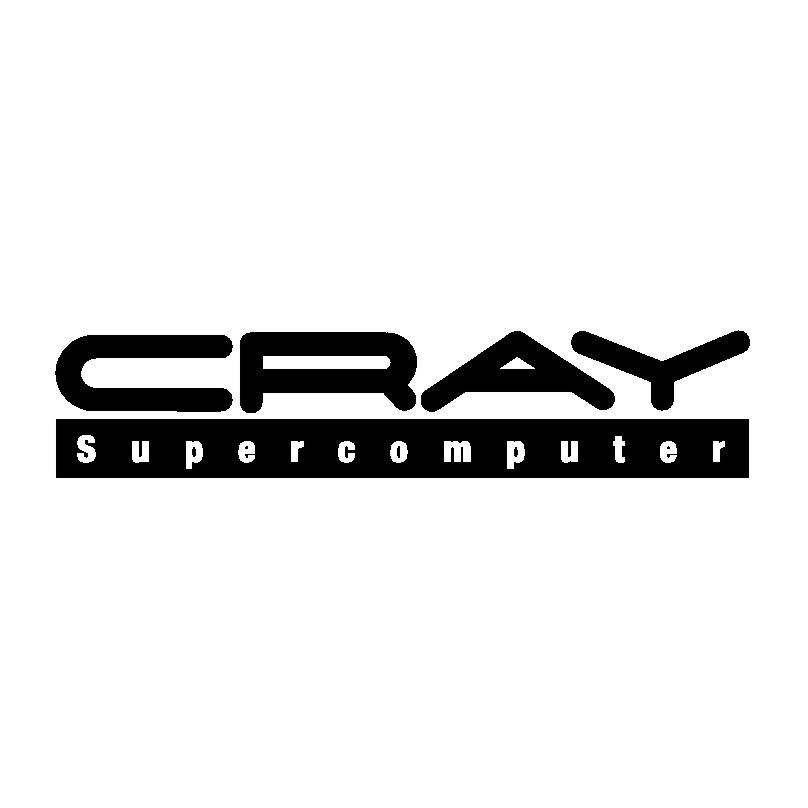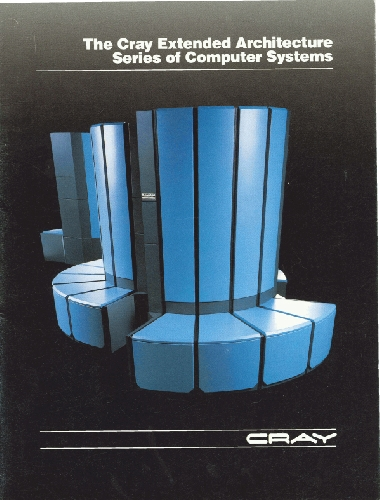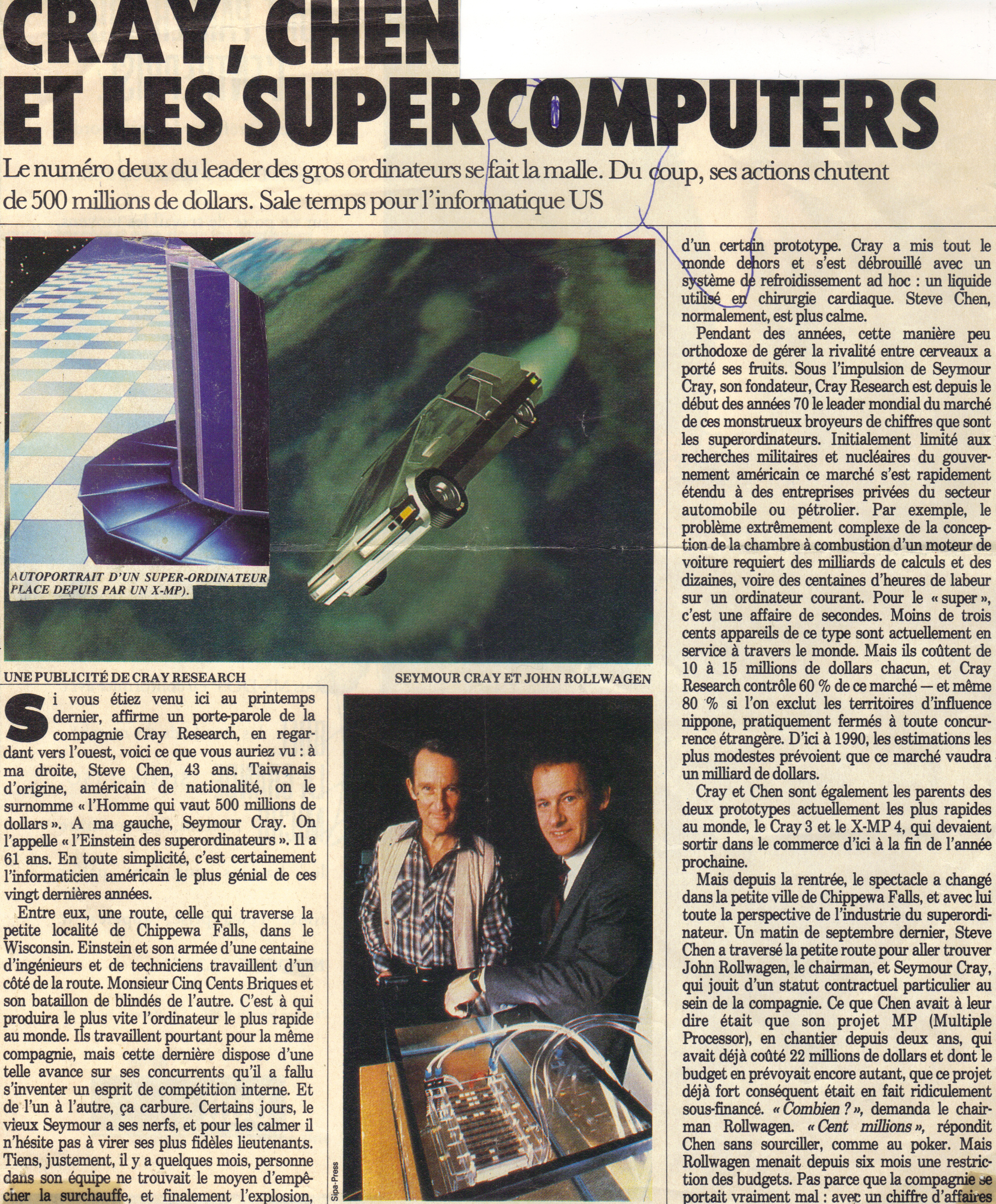In 1982 a CRAY mainframe computer had 8MB of memory at a cost of \$7,000,000. For TRON, each rendering required 18MB of memory, so even on a CRAY, the animators could not have viewed a single frame. So for whatever computer they used, they had to render one scan line at a time, and could not see the results of their efforts until the scan lines were combined on to film.

## Computer graphics made by Cray Supercomputer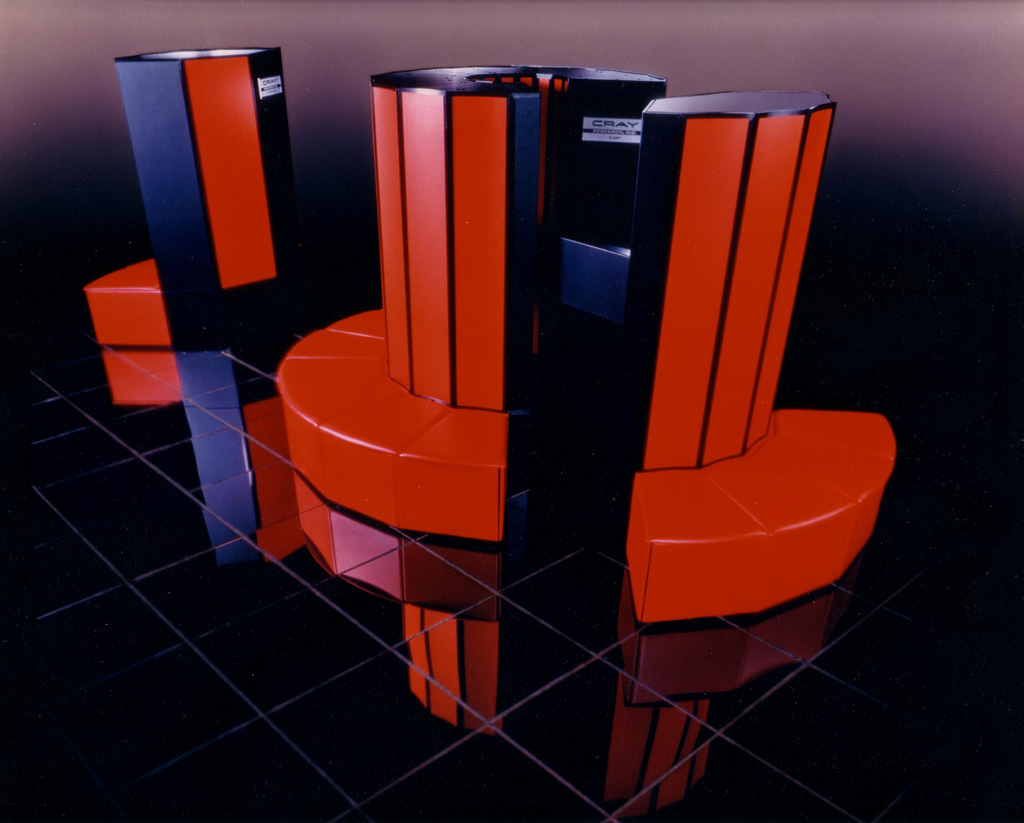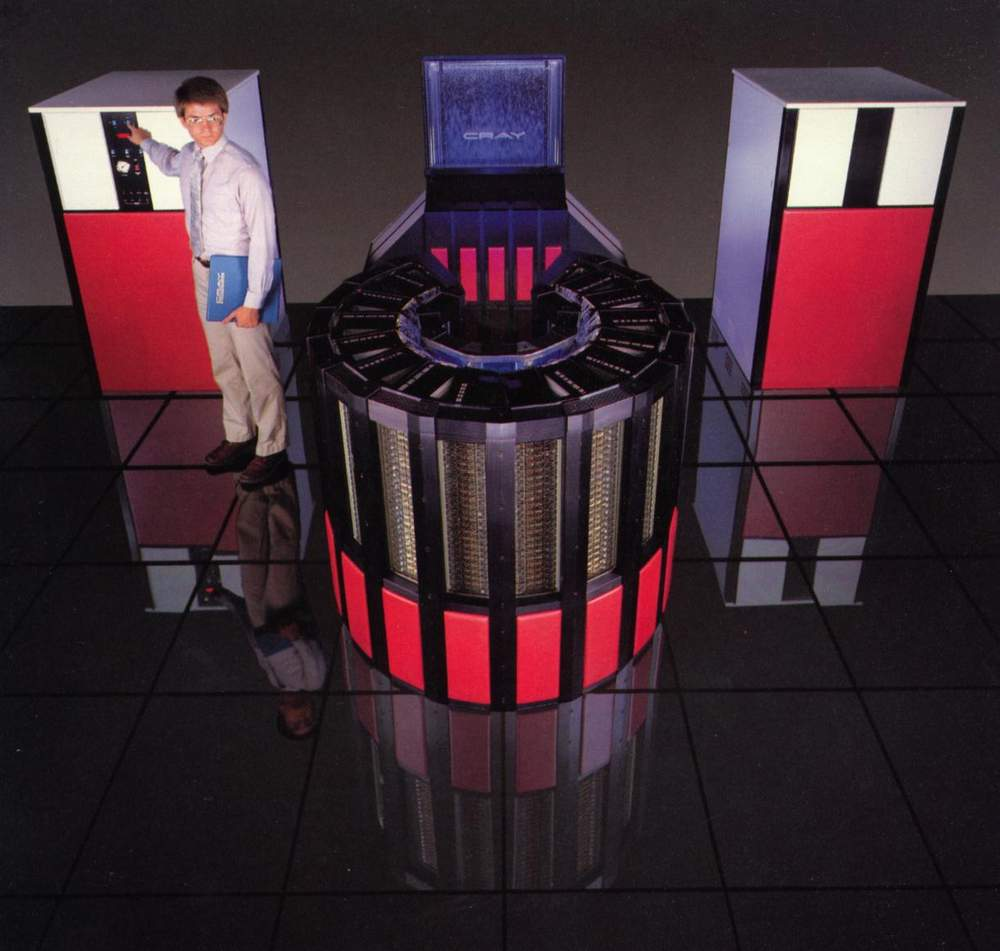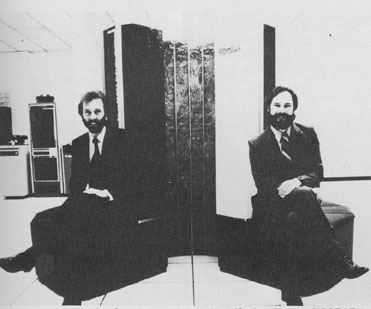## Early Alias team FUTURE AUTODESK MAYA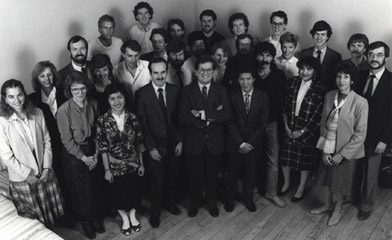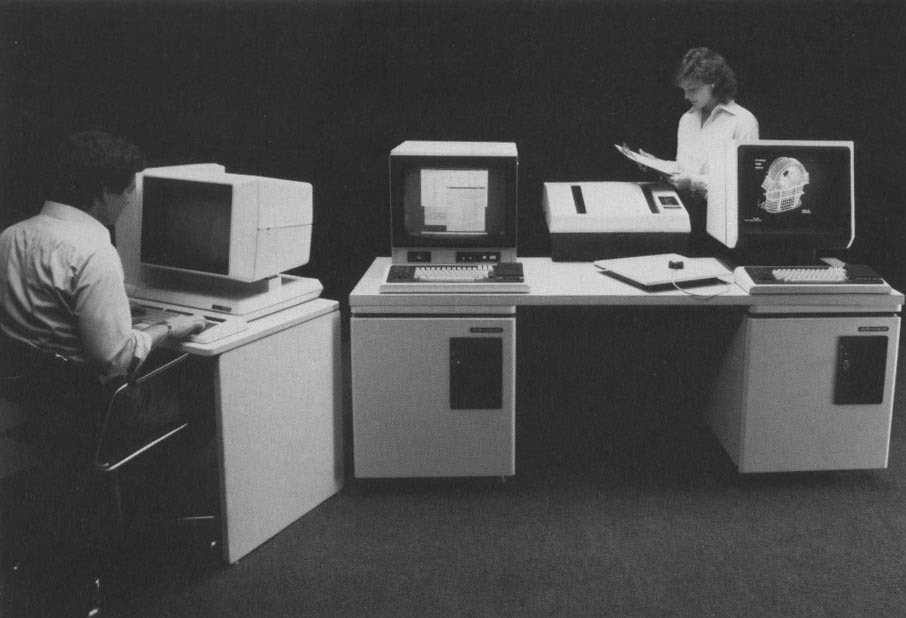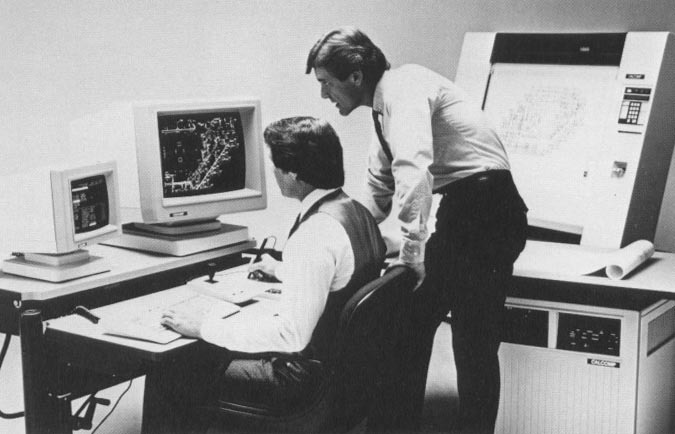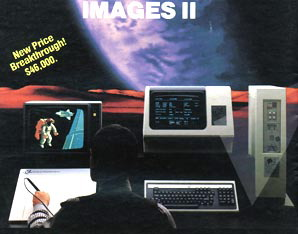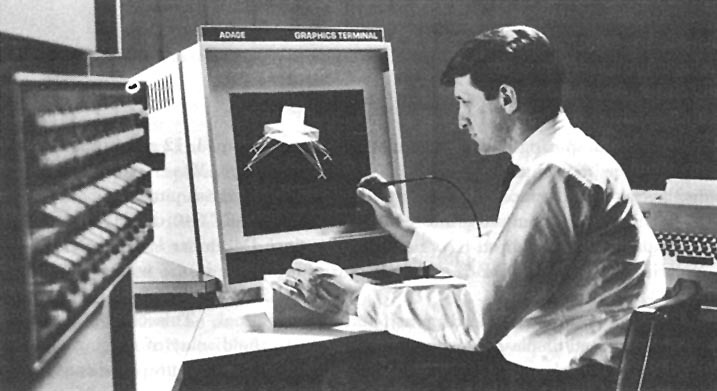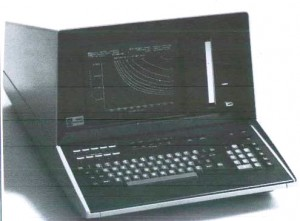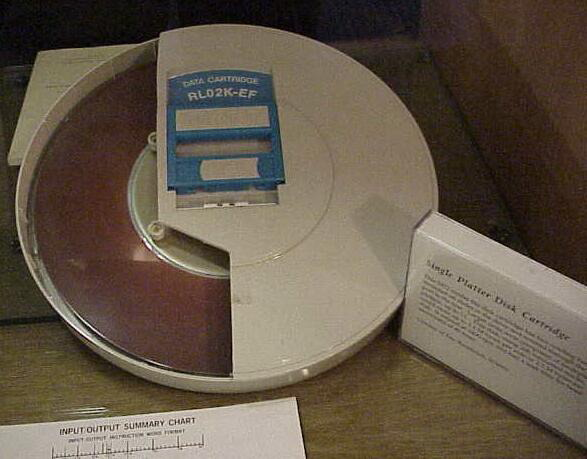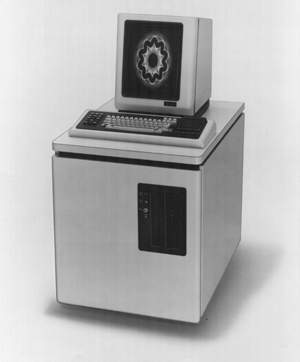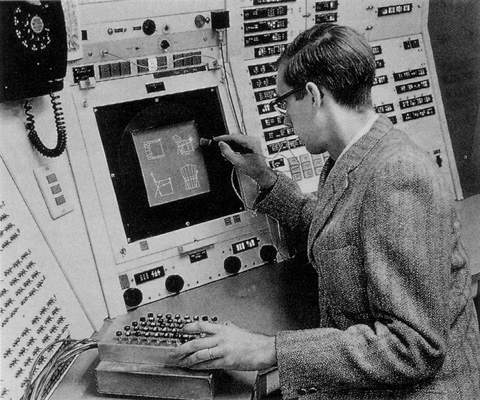## VAX_11_750 SUPERCOMPUTER

Speed : 1 MIPS

Memory Type : 4K MOS RAM

Memory Size : 1 MB + Memory Width 32-bit

Cost : \$120,000 - \$160,000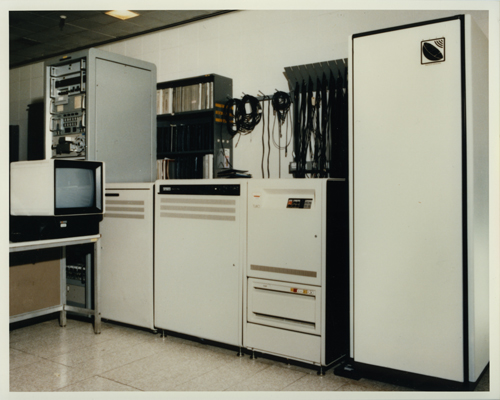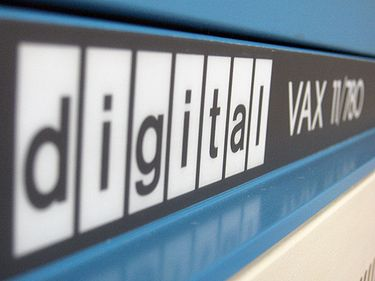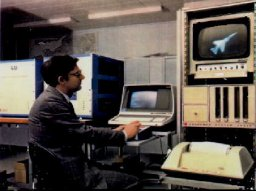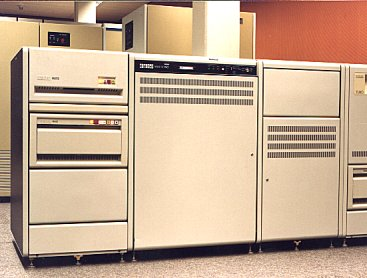## MIPS - Million instructions per second

Million instructions per second (MIPS) is an older, obsolete measure of a computer's speed and power, MIPS measures roughly the number of machine instructions that a computer can execute in one second. However, different instructions require more or less time than others, and there is no standard method for measuring MIPS. In addition, MIPS refers only to the CPU speed, whereas real applications are generally limited by other factors, such as I/O speed.

A machine with a high MIPS rating, therefore, might not run a particular application any faster than a machine with a low MIPS rating. For all these reasons, MIPS ratings are not used often anymore. In fact, some people jokingly claim that MIPS really stands for Meaningless Indicator of Performance.

Despite these problems, a MIPS rating can give you a general idea of a computer's speed. The IBM PC/XT computer, for example, is rated at Ľ MIPS, while Pentium-based PCs run at over 100 MIPS.

For all the above reasons, MIPS ratings are not as often used today because of the development of other processor benchmarks that give a more accurate rating of a computer's performance. However, the MIPS measurement has been used by many computer manufacturers like IBM to measure the "cost of computing." The value of computers is determined in MIPS per dollar. Interestingly, the value of computers in MIPS per dollar has steadily doubled on an annual basis for the last couple of decades. As an example of the advancement of CPU speed over the last two decades, The Motorola 68000 processor 1979 ran at 1 MIPS at 8 Mhz in the year 1979 as compared to the Intel Core 2 X6800 processor which ran at 27,079 MIPS at 2.93 Ghz in 2006.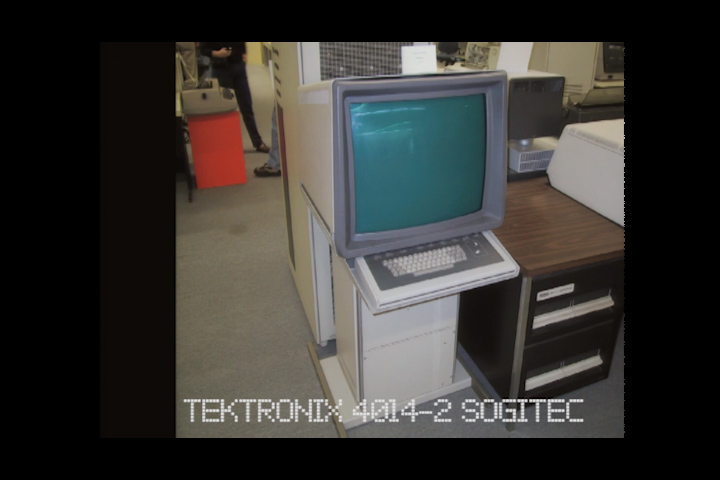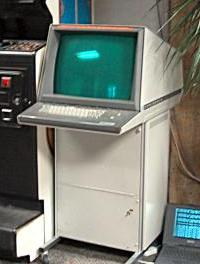` Selected Tek Storage Tube Terminals`
` `
```
```

The 80s music & Fx video production with the BOSCH FGS-4000 ( Dire Straits)

## SUPERCOMPUTERS WORLD

DGSN COMPUTER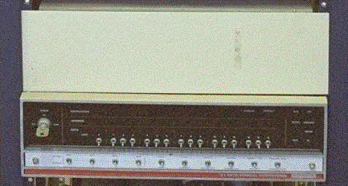nova840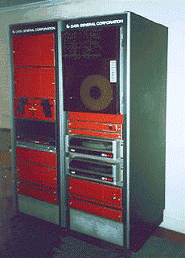nova4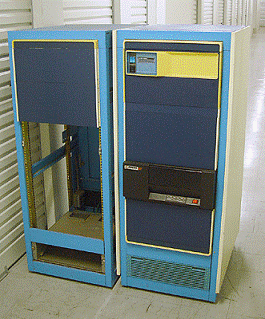eclipse-s130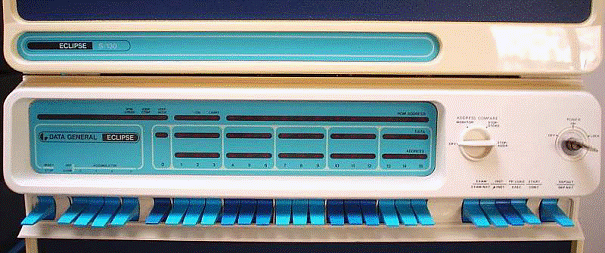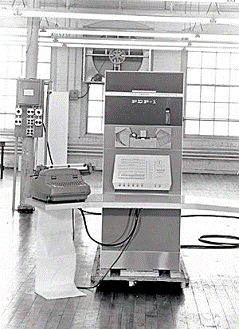pdp4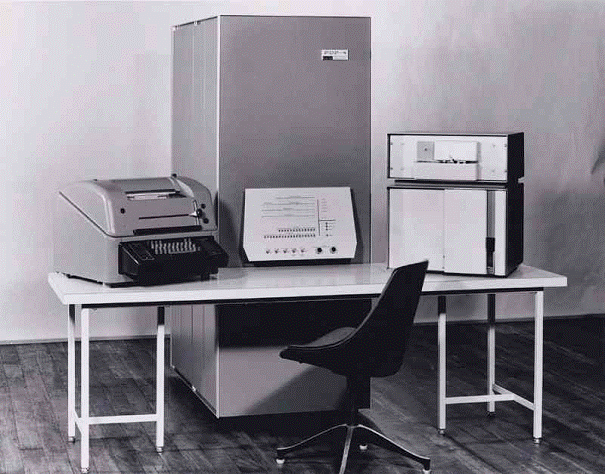pdp5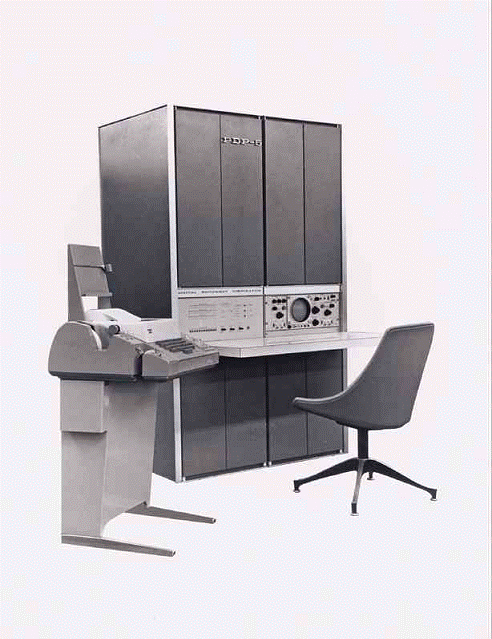pdp6.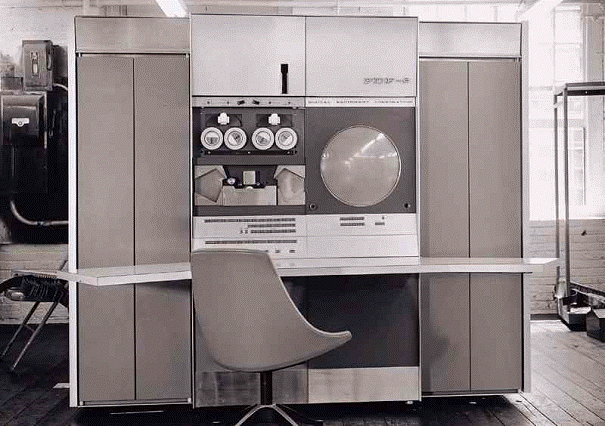pdp8i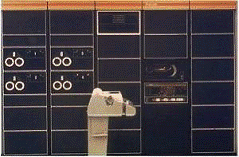pdp8e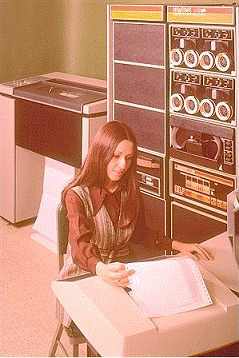vt78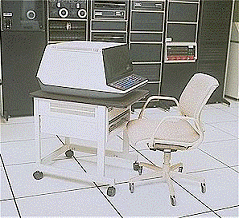pdp10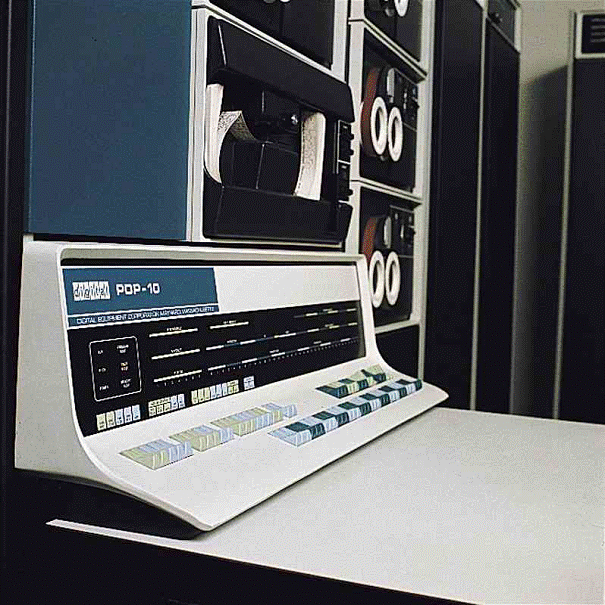decsystem_10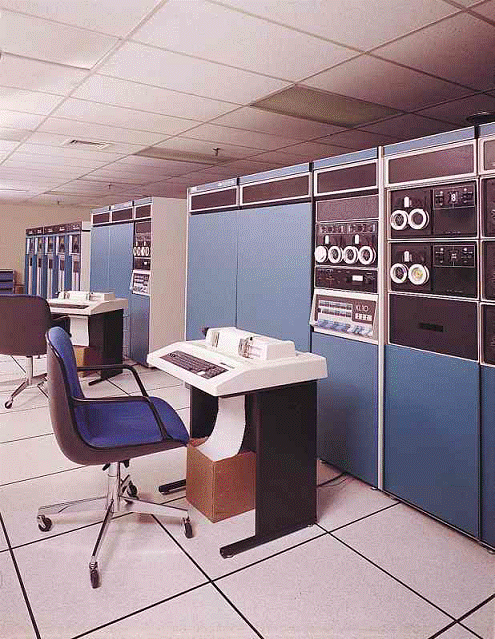decsystem_20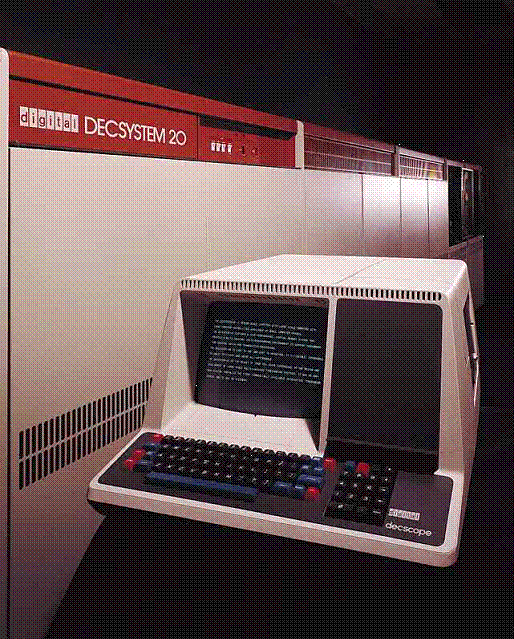/pdp11_20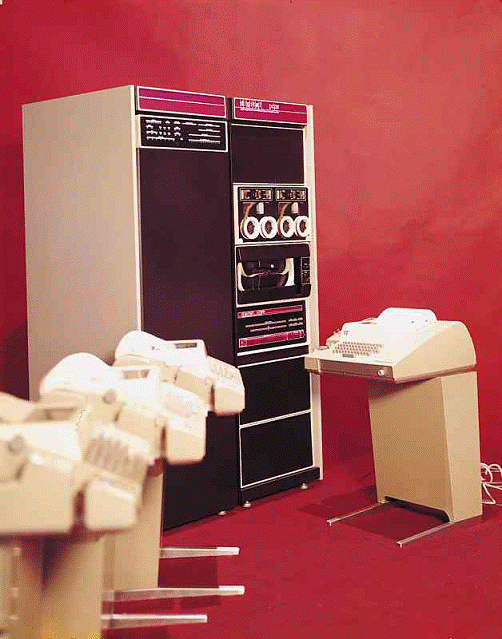/pdp11_45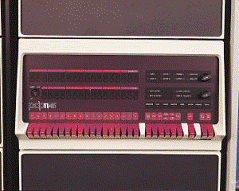pdp11_05pdp11_40pdp11_34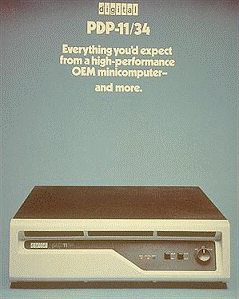pdp11_70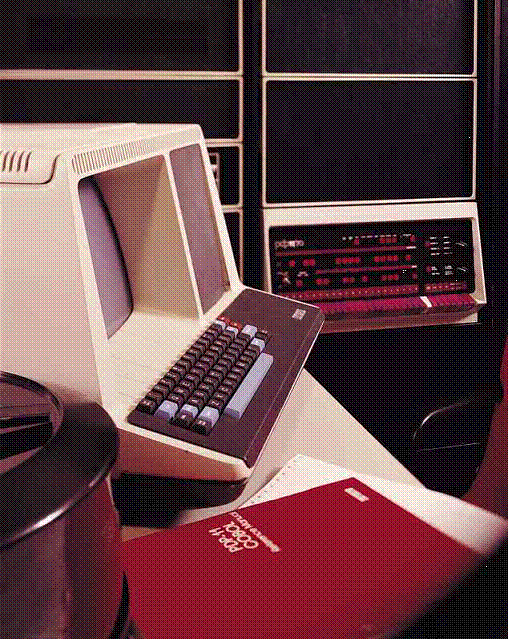/pdp11_60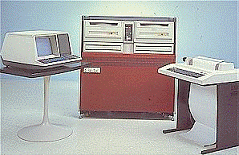pdp11_24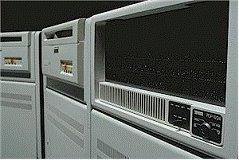pdp11_44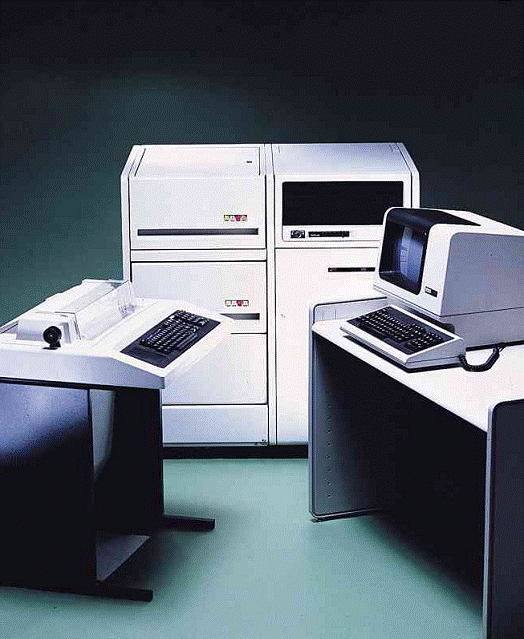pdp12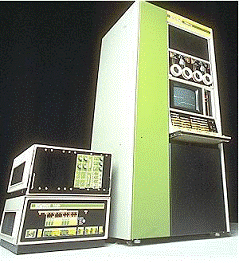pdp15

>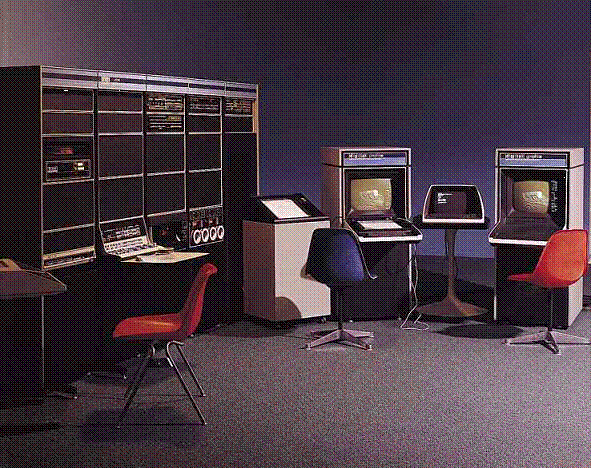ss="MsoNormal">DGSN COMPUTER

## PERKIN HELMER 3200 MPS USED BY NASA AND OUR STAR SOGITEC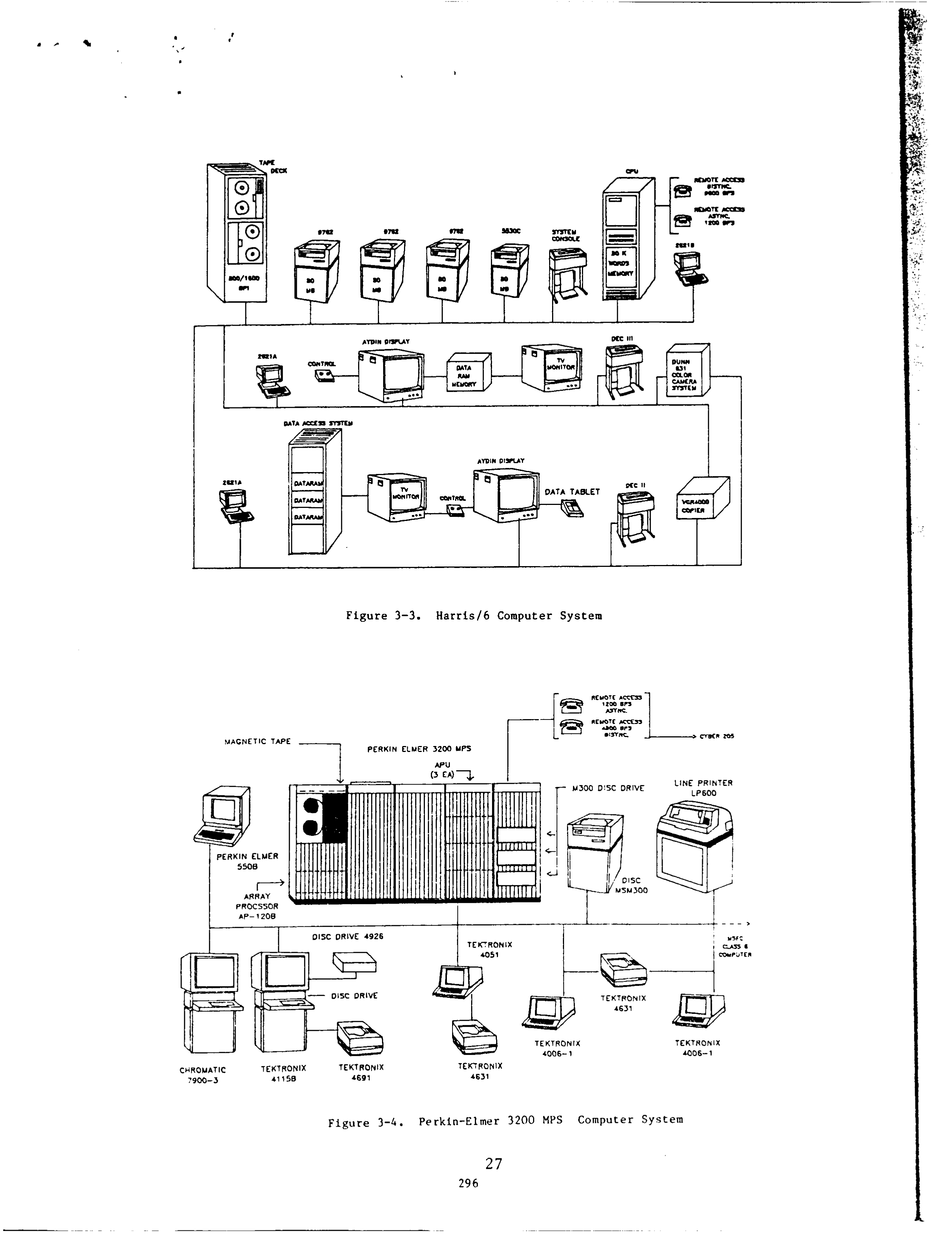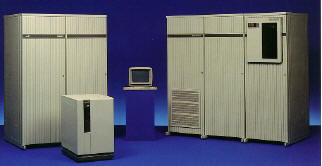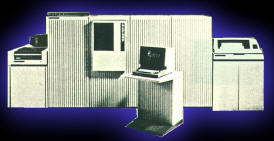INTERACWJE INFORMATION PROCESSING FOR THE ATMOSPHERIC SCIENCES DIVISION AT NASA’s MRSWL SPACE PLIGHT CENTER

I. INTRODUCTION This paper d e s c r i b e s t h e i n t e r a c t i v e comp u t e r r e s o u r c e s u t i l i z e d by t h e Atmospheric Sciences Division (ASD) at NASA’s Marshall Space Flight Center (MSFC). The primary focus here is t o d e s c r i b e t h e o v e r a l l d a t a p r o c e s s i n g a c t i v i t i e s . a v a i l a b l e computer resources, data management, and s o f t w a r e a p p l i c a t i o n s . Highl i g h t i n g its effectiveness as a useful i n t c r a c - t i v e r e s e a r c h t o o l . a demonstration of the ASD computer f a c i l i t y w i l l be given a t t h i s conference . v i a remote a c c e s s t o MSFC’s mainframe computers u s i n g both IBM PC/AT and Apple 111 microcomputer workstations.

2. PROGRAM OVERVIEW The Atmospheric Sciences Division is involved in the design, t e s t i n g , data analysis and research r e l a t e d t o new remote s e n s i n g i n s t r u - ments f o r f u t u r e s a t e l l i t e , a i r c r a f t , Spacelab, and space s t a t i o n missions. These a c t i v i t i e s include the Doppler Lidar System, Lightning Mapper, High Resolution Interferometer Sounder, Ceophysical F l u i d Flow C e l l , M u l t i s p e c t r a l Atmospheric Mapping Sensor, Space Shuttle Main Engine, Satell i t e P r e c i p i t a t i o n and Cloud Engineering, Earth O b s e r v a t i o n M i s s i o n , Advanced M i c r o w a v e P r e c i p i t a t i o n Radiometer. and t h e Mesoscale Analysis and Space Sensor programs.

In conjunction with the above, i n t e r a c t i v e information processing is employed in t h e a r e a s of m u l t i s p e c t r a l image p r o c e s s i n g ( v i s i b l e , i n f r a r e d , m i c r o w a v e ) , a t m o s p h e r i c modeling ( d e n s i t y , c i r c u l a t i o n , t u r b u l e n c e , f l u i d d y n a m i c s ) , d a t a management ( d o p p l e r l i d a r , l i g h t n i n g , s a t e l l i t e imagery, s o l a r a c t i v i t y , atmospheric d a t a s e t s , f l i g h t experiment d a t a , e t c . ) , real-time s a t e l l i t e data i n g e s t , i n t e r a c - t i v e data display and analysis, and computational f luid dynamics.

3. COMPUTER RESOURCES TO s a t i s f y e v o l v i n g d a t a p r o c e s s i n g requirements, the e x i s t i n g ASD computer f a c i l i t y has continued t o be upgraded and i t s computer r e s o u r c e s expanded by i n t e g r a t i n g i n t e r a c t i v e microcomputer workstations. Through these work- Laura UacLean Paul Meyer Atmospheric Sciences Division Systems Dynamics Laboratory Marshall Space Plight Center, AL 35812 John S. Hickey Shogo Karitani Atsuko Computing I n t e r n a t i o n a l Huntsville, AL 35801 Karen Parker Karen Payne New Technology, Inc. Huntsville, AL 35806 s t a t i o n s , access is provided t o the ASD f a c i l i t y , the Engineering Analysis and Data System (EAIS), and to o t h e r a c c e s s i b l e computers.

3.1 ASD Computer System The e x i s t i n g ASD computer system c o n s i s t s of t h r e e primary computers (Hewlett-Packard IOOOF, Harris/6, Perkin-Elmer 3200 MPS) and ass o c i a t e d p e r i p h e r a l s along with I B M PC/AT and APPLE 111 microcomputer workstations t h a t provide over 30 ASD s c i e n t i s t s with a wide range of unique c a p a b i l i t i e s f o r processing and displaying data (see Figure 3-1).

The Hewlett-Packard (W-1OOOF) is u t i l i z e d for management of large volumes of conventional and s a t e l l i t e d e r i v e d m e t e o r o l o g i c a l d a t a , analysis and graphical d i s p l a y , and general purpose computing. The HP-1000F system c o n s i s t s of 1.25 Mb of main memory, 575 Mb disk storage, and operates under RTE-6/VM Virtual Memory Operating System with S e s s i o n Monitor. A hardware conf i g u r a t i o n of the HP-1000F system is provided in Figure 3-2.

The Harris/6 McIDAS (Man-computer Interact i v e Data Access System) is connected t o the IBM McIDAS system a t the Space Science and Engineering Center (SSEC) a t the University of Wisconsin- Madison, which allows for convenient access and analysis of real-time d a t a , s a t e l l i t e and convent i o n a l r e s e a r c h d a t a b a s e s , and p r o v i d e s f o r graphical display/animation of space image data. The Harris/6 McIDAS system c o n s i s t s of 0.25 Mb of main memory, 320 Mb disk storage. and operates under Modified DMS. A d e t a i l e d hardware conf i g u r a t i o n of the Harris/6 McIDAS system is shown in Figure 3-3.

The Perkin-Elmer (P-E 3200 MPS) is u t i l - ized primarily f o r numerical modeling and large number crunching tasks. It is a multiprocessor system c o n s i s t i n g of three a u x i l i a r y processing u n i t s (APU‘s), 16 Mb of i n t e r l e a v e d main memory, 1200 Mb d i s k s t o r a g e , and operates under OS/32 Revision 8.1 with Multi-Terminal Monitor (MTH). An array processor (FPS AP-12OR) is a l s o attached t o t h i s system f o r off-loading CPU bound jobs. A d e t a i l e d hardware c o n f i g u r a t i o n of the P-E 3200 MPS system is shown i n Figure 3-4.

HARRIS/S TERMINAL IA mr .o11~ nunm m HE'NLETT PACKARD 1 OOOF TEXTRONIX CHROMATIC LOCAL AND REMOTE WORKSTATIONS

Figure 3-3. Harris16 Computer System 1 PERKIN ELMER 5MfJ r-+ ARRAY PRQCSSOR AP- 1200 I I DISC DRIVE 4926 L.-., TCKTRQNIX TE KTRONIX TEKTRQNIX 4006- 1 1006-1 ChROMATlC TEKTRONIX TEKTRONIX TEKiRQNlX 7900-1) 4 1 1 5 0 4691 4631 Figure 3 - 4 . Perkin-Elmer 3200 MPS Computer System 27 296

.

3.2 Engineering Analysis 6 Data System The EADS is a c l a s s s i x supercomputer f a c i l i t y t h a t is being implemented at MSFC. It c o n s i s t s of a high speed vector processor, a vast amount of memory and on-line storage, monitor and c o n t r o l d e v i c e s , an i n t e r a c t i v e t e r m i n a l subsystem, common output devices, a Local Area Network (LAN) and v a r i o u s g r a p h i c s o f t w a r e packages. A t t h i s time, the EADS is only part i a l l y o p e r a t i o n a l w i t h f u l l operations planned f o r e a r l y 1986 (see Figure 3-5). The High Speed Vector Processor (HSVP) is a CRAY X-MP/44 computer, c o n s i s t i n g of f o u r p a r a l l e l CPU’s with 4 megawords of shared memory and dedicated d i s k s t o r a g e of 8.4 Gb. The HSVP has an overall performance which is typically ten times t h a t of the CRAY-1.

The Front End Processor (FEP) is an IBM 1954 with the MVS/XA operating system and is comprised of two IBM 1951 KX processors, each with 32 Mb memory and lOGb of dedicated disk storage and 15 Gb of disk storage s h a r e d by an IBM 4381-2 p r o c e s s o r w i t h 16 Mb memory and 2.5 Gb o f d e d i c a t e d d i s k s t o r a g e . These machines are redundantly interconnected in order t o avoid a s i n g l e point o€ f a i l u r e . The IBM 4381-2 is r e s p o n s i b l e f o r communications c o n t r o l and image processing. Two IBM 3725 c o m u n i c a t i o n s c o n t r o l l e r s handle most of the terminal t o host communications.

Three image processing workstations are being i n s t a l l e d c o n s i s t i n g of t h e f o l l o w i n g elements: a VAX 11f730 with 2 Mb memory, 456 Mb disk drive, Int e r n a t i o n a l Imaging Systems (LIS) model 575 hardware and software, Tektronix hardcopy u n i t , 112‘’ and 314“ b r o a d c a s t q u a l i t y V C R ‘ s , and a Dunn model 635 camera. One of the image workstations is equipped with an Optronics d i g i t i z i n g camera system as both an i n p u t and o u t p u t device. Emulation s o f t w a r e is a v a i l a b l e t o u t i l i z e the image workstation as a McIDAS terminal, and furt h e r software development w i l l allow image processing c a p a b i l i t i e s in both McIDAS and IIS modes. For l a r g e amounts of on-line f i l e storage, a MASTOR model M860 unit can s t o r e 195 Cb of data on t a p e c a r t r i d g e s . This system c o n s i s t s of an IBM 4341 computer with several d i s k d r i v e s and is a c c e s s i b l e from the FEP and IBM 4381-2.

The EADS network provides various output devices including an NCR 5330 microfilm machine capable of both FR-80 and hardcopy output, tvo IBM 4248 p r i n t e r s and a XEROX 9600 laser p r i n t e r , a c o l o r f i l m p r o c e s s o r t o g e n e r a t e movies and viewgraphs, and several other RJE p r i n t e r s and terminals located throughout MSFC.

3.3 Communications The ASD computer system and EADS can both be accessed from a workstation via a HYBERbus Loc a l Area Network (9600 baud). In a d d i t i o n , s e l e c t i v e access t o each of the three ASD computers is provided by a 9600 baud ASD f a b r i c a t e d ’patch panel‘ network. The ‘patch panel’ allows up t o 60 users to simultaneously route a works t a t i o n d i r e c t l y to the desired ASD computer, Remote i n t e r a c t i v e access to the ASD computer system and EADS is provided by connecting t o MSFG’s Develcon d a t a s w i t c h v i a 1200 baud asynchronous RS-232 l i n e s with XONIXOFF protocol. For the ASD system, one port each is connected t o the Harris16 and P-E 3200 KPS. The HP-1000F u t i l i z e s t h e o t h e r two l i n e s with t h e a d d i t i o n a l option of using ENQIACK protocol. Remote access t o the EADS is provided through a number of data switch l i n e s i n t o the system.

The HP-1000F s u p p o r t s t h e DS-1000 communications network which provides for computer to computer d a t a t r a n s f e r using a d i a l - u p 1200 baud l i n e . The ASD u t i l i z e s the DS-1000 network t o receive real-time TVA p r e c i p i t a t i o n data for immediate processing and analysis. The Harris16 system also provides remote c o m m u n i c a t i o n t h r o u g h a DDS 9 6 0 0 b a u d bisynchronous communication l i n e with t h e IBM 4381 McIDAS a t the SSEC. The l i n e is used as a computer to computer data l i n k t o t r a n s p o r t case study data sets. These d i g i t a l and t e x t f i l e s can be t r a n s f e r r e d i n e i t h e r d i r e c t i o n along t h i s DDS c i r c u i t . In addition, t h i s l i n e is used t o d i r e c t l y connect a McIDAS terminal t o the SSEC, a l l o w i n g f o r r e a l - t i m e s u p p o r t of ASD f l i g h t experiments. The P-E 3200 MPS system o f f e r s an RJE conn e c t i o n v i a a 4700 baud bisynchronous modem driven by HASP protocol. S c i e n t i s t s a r e using t h i s l i n k t o access NASA’s Cyber 205 located at Goddard Space Flight Center. Figure 3-5. EADS System Con€iguration 28

3.4 ASD Workstations The IBM PC/AT and APPLE I11 microcomputers are being u t i l i z e d as workstations t o allow the ASD s c i e n t i s t s t o access a v a i l s b l e NASA/HSPC computers from t h e i r o f f i c e or remotely (see Section 3.3 Communications). A workstation can be connected to one of t h e t h r e e ASD computers, the EADS system, or u t i l i z e d i n a standalone mode, a l l w i n g the scientist to i n t e r a c t i v e l y analyze and display various data. The APPLE 111 workstations are i n t e g r a t e d i o t o t h e ASD s y s t e m w i t h t h e f o l l o w i n g c a p a b i l i t i e s : 256 Kb memory, letter quality and dot matrix p r i n t e r , color p l o t t e r , color hi-res m o n i t o r , B6W monitor. Novation 212A or Hayes Smart Modem (1200 baud), real-time clock, and 5 Mb p r o f i l e h a r d d i s k . S o f t w a r e h a s been developed t o allow the APPLE 111 workstation to b e u s e d as a H e w l e t t - P s c k a r d t e r m i n a l w i t h graphics c a p a b i l i t y and future development is underway t o emulate the McIDAS graphics terminal.

Various software packages are a l s o provided for the APPLE 111's t o be used in a standalone mode including PASCAL Compiler and APPLE-Writer. The IBM PC/AT workstations are integrated i n t o t h e ASD system with t h e f o l l o w i n g capa b i l i t i e s : 512 Kb Memory, letter q u a l i t y and dot matrix p r i n t e r , IBM enhanced g r a p h i c s d i s p l a y monitor, and 20 Hb hard disk. Software is being developed t o allow the IBM PC/AT workstation to be used as an Hewlett-Packard graphics terminal and a McIDAS graphics terminal. Various software packages are a l s o provided f o r the IBM PC/AT's to be used in a s t a n d a l o n e mode i n c l u d i n g TURBO PASCAL, dBase 111, WORDSTAR 2000, MicroSoft FORTRAN, CROSSTALK, DISPLAWRITE and others.

3.5 McIDAS Terminal The McIDAS t e r m i n a l is a s p e c i a l i z e d m e t e o r o l o g i c a l w o r k s t a t i o n w i t h a number of powerful a t t r i b u t e s developed s p e c i f i c a l l y f o r d i s p l a y i n g meteorological satellite and convent i o n a l data. A powerful aspect of the McIDAS terminal is its a b i l i t y t o o v e r l a y g r a p h i c s on a satellite image. In t h i s way, plotted or cont o u r e d m e a s u r e m e n t s f r o m t h e f i e l d can be v i s u a l l y r e l a t e d via animation c a p a b i l i t i e s t o the s a t e l l i t e image. An a d d i t i o n a l feature is the red-green s t e r e o display of imagery which allows f o r t h r e e - d i m e n s i o n a l d i s p l a y c a p a b i l i t y . Also, color hardcopies are a v a i l a b l e using the Dunn 605 camera, black and white hardcopies using the Honeywell v i s i c o r d e r , and v i d e o r e c o r d i n g using the VCR's.

The t e r m i n a l c o n s i s t s of a keyboard and CRT monitor with f u l l 'video' d i s p l a y , a memory u n i t , p r i n t e r , j o y s t i c k s , and data t a b l e t . The memory d e v i c e can store 32 image frames, 16 graphic frames and has the c a p a b i l i t y of expansion with a d d i t i o n a l memory boards. Users of t h e McIDAS t e r m i n a l may e n t e r t h e i r commands from e i t h e r a standard keyboard or v i a t h e d a t a t a b l e t . The j o y s t i c k s may be used f o r c u r s o r and c o l o r enhancement c o n t r o l . An added c a p a b i l i t y is t h a t a l l keys a r e f u l l y programmable a l l o w i n g t h e u s e r t o e x e c u t e a variety of commands with a s i n g l e keystroke.

4. DATA WAGEPIENT It is necessary t h a t v a r i o u s experiment data be e a s i l y a c c e s s i b l e throughout the e n t i r e ASD computer system in order to accomplish the r e q u i r e d r e s e a r c h . T h e r e f o r e , a few standard formats have been set f o r t h to r e s t r u c t u r e t h e i n - c o m i n g d a t a which a r r i v e s from numerous s o u r c e s and in v a r i o u s formats. S e v e r a l ASD developed data management u t i l i t y routines reside on the HP-1000F and are u t i l i z e d to reformat the data and generate random sccess d i s c f i l e s on the HP-1000F or magnetic tape output which allows the d a t a t o be t r a n s f e r r e d between t h e t h r e e ASD computers. F i g u r e 4-1 shows t h e o v e r a l l d a t a flow throughout the ASD computer system.

There are f o u r b a s t c data types currently being p r o c e s s e d on t h e HP-1000F: Soundings (Rawinsonde, S a t e l l i t e ) . Single Level (Surface, Cloud Winds, LLP, P r e c i p i t a t i o n ) , Grids (same a s S i n g l e L e v e l ) , and Images ( S a t e l l i t e , Radar). The HP-1000F data management s o f t w a r e c o n v e r t s the various data and creates a 'random access' disk f i l e , thus making the data e a s i l y accessible t o numerous ASD a n a l y s i s and d i s p l a y software packages. Associated with each 'random access' data f i l e is a ''Documentation'' f i l e which desc r i b e s the data set and a " L a t i t u d e / L o n g i t u d e " f i l e which provides information for graphically p l o t t i n g the s t a t i o n locations. In addition, a "Directory" f i l e e x i s t s f o r each of t h e d a t a types, which contains the f i l e names, parameter indexes, t i m e periods, dates, titles, and other information.

The Harris/6 o f f e r s the s c i e n t i s t a visual means of studying s a t e l l i t e , radar, or convent i o n a l d a t a in an i n t e g r a t e d manner. All of the d a t a t h a t comes i n t o t h e McIDAS system is of t h r e e b a s i c forms: Images, Grids, and 'Station Data Sets' (SDS) f i l e s . McIDAS images and g r i d s are r a t h e r s t r a i g h t forward, two-dimensional data s t r u c t u r e s . The SDS s t r u c t u r e is one in which there a r e any number of measurements made at one location at a given t i m e .

C o n v e n t i o n a l b a l l o o n , surface, s a t e l l i t e temperature and moisture soundings, and lightning strokes are examples of data t h a t are put i n t o SDS f i l e s . Radar data has been put i n t o both the image and the grid format. Standard objective analysis software routines transform SDS data t o t h e g r i d s t r u c t a r e , u s u a l l y f o r the contour a p p l i c a t i o n s . The grid can then be p u t i n t o an image format f o r display. Transformation in the reverse d i r e c t i o n from t h e Image s t r u c t u r e t o t h e other s t r u c t u r e s is usually done with a spec i a l i z e d r e s e a r c h g o a l in mind, and algorithms a r e q u i t e dependent upon the s p e c i f i c goal.

The P-E 3200 MPS is used to generate vast amounts of d a t a by s o f t w a r e which s i m u l a t e s v a r i o u s t y p e s of a t m o s p h e r i c phenomenon. Res e a r c h s c i e n t i s t s use t h i s s i m u l a t e d d a t a t o c o r r e l a t e t h e i r mathematical models to actual data. The model output is stored i n contiguous data files which a r e accessed both r,indomly and sequentially. NCAR graphics output is stored in 'metacode' format which is device independent. Data is e a s i l y t r a n s f e r r e d to and f r o m the P-E 3200 MPS using standard P-E copy u t i l i t i e s .

29 298 HIRRIS/E O/S: YWlFlED DYS 24 BIT WORD zsue UCY XOUB os REYIWCH DATA INPUTS: --IIICITIZER --Ysfc,’iau Q .Cy1 DATA --REYW*BE DISC CARTRIDGE -ARCHIYE *“T‘ .Q / Figure 4-1. ASD System Data Flow

5. ASD SOFTWARE APPLICATIONS Various a p p l i c a t i o n programs and models are e x e c u t e d on a d a i l y b a s i s by s c i e n t i s t s u t i l i z i n g the ASD computer system. Specific app l i c a t i o n s are performed on the ASD computer that best s a t i s f i e s the requirements of the program. The HP-1000F computer system is h e a v i l y u t i l i z e d t o analyze and g r a p h i c a l l y d i s p l a y large volumes of atmospheric data by the use of an int e r a c t i v e analysis and display software package (AVEBO). This package is a *menu driven‘ set of F o r t r a n programs which p r o c e s s t h e four basic t y p e s of d a t a (Sounding, S i n g l e L e v e l , Grid, Image) and generates various outputs based upon t h e user’s s e l e c t i v i t y . By u s i n g t h e AVEBO programs, atmospheric data may be displayed i n various forms such as Skew T p l o t s , parameter value and p r o f i l e plots, s t a t i o n and parameter base map p l o t s , wind v e c t o r and wind p r o f i l e p l o t s , w i n d b a r b p l o t s , g r i d p a r a m e t e r contouring, printed grid p r o f i l e , shaded printed images, and colored image displays/animation (see Figure 5-1 1.

The AVE80 program w i l l prompt the user for v a r i o u s i n t e r a c t i v e i n p u t s f o r q u a l i f y i n g the desired data options. The user may process data on a s i n g l e case basis or select multiple cases. The u s e r t h e n may s e l e c t t h e d e s i r e d d a t a p a r a m e t e r s s u c h as d a t a t y p e , d a t a base, time periods, s t a t i o n numbers, l a t i t u d e , l o n g i t u d e , p r e s s u r e l e v e l s , etc. F i n a l l y t h e u s e r may s e l e c t t h e output device , l i n e s t y l e s , colors and s e v e r a l o t h e r options depending on the output desired. Output devices a v a i l a b l e to the user i n c l u d e : HP X-Y c o l o r p l o t t e r s , HP g r a p h i c s p r i n t e r / p l o t t e r , HP T h i n k j e t p r i n t e r , HP Laser Jet p r i n t e r , color monitors, and APPLE LIT and IBM PC/AT workstations. Several high-level programs e x i s t on the McIDAS t o permit application of sophfstfcated algorithms to image data. One such program uses many images a t d i f f e r i n g s p e c t r a l wavelengths to reproduce v e r t i c a l atmospheric temperature and moisture soundings. Another program uses several images for an animated sequence f o r the purpose of c a l c u l a t i n g tracked winds. Rainfall estimat i o n algorithms have been developed which u t i l i z e infrared and microwave images. These techniques aid in determi ni ng preci p i t a t i on c h a r a c t e r i s t f cs over both land and oceans. Figure 5-2 shows example outputs from the McXDAS system.

The P-E 3200 NPS system is used primarily f o r e x e c u t i n g a t m o s p h e r i c p h y s i c s and f l u i d dynamics r e s e a r c h models of high CPU bound a c t i v i t y . The P-E a l s o s u p p o r t s t h e NCAR g r a p h i c s package w i t h metacode t r a n s l a t o r s f o r Tektronix terminals and FR80 microfiche output. Color g r a p h i c s c a p a b i l i t y is a v a i l a b l e on the Tektronix 4115B and Chromatics t e r m i n a l , which support the i n t e r a c t i v e research of the Doppler Radar/Lidar a c t i v i t i e s , s p e c i f i c a l l y using t h e NEXRAD software from the National Severe Storms Laboratory, The atmospheric physics models include the M u l t i s p e c t r a l Atmospheric Napping Sensor (HAMS) which measures a t m o s p h e r i c , c l o u d and s u r f a c e c h a r a c t e r i s t i c s at very f i n e h o r i z o n t a i resolut i o n with spectral imagery ( s e p a r a t e v i s i b l e and i n f r a r e d s p e c t r a l bands) to map the 4-D atmospheric temperature, m o i s t u r e and cloud f i e l d s . Other models run on the P-E fnclude t h e Limited Area Mesoscale P r e d i c a t i o n System (LAMPS), P i e l k e ‘ s , Cloud Wind and t h e South Dakota 2-D cloud model.

Space S h u t t l e Main Engine (SSME) and S p a c e l a b p r o j e c t s use t h e P-E system p r f m r i l y f o r computational and geophysical f l u i d dynadce modeling. The s c i e n t i s t s execute CW’s PHOENICS code which allows them to simulate flows and generate graphics output. Figures 5-3 and 5-4 show example outputs from the P-E system. 30 299 .* I' * * . * L 7%'..,.. -. 1.' i 2 ,-J .... ... .. Figure 5-1. HP-IOOOP AVE80 Program Outputs Figure 5-2. Harris/6 McIDAS System Outputs 31 300 ,. . C

6. SUMMARY - IABATICH EA~NGRA TE 4.5 KM Figure 5-3. P-E 3200 LAMPS Model Output Figure 5-4. P-E 3200 NEXRAD Model Output 32 30 1 I n summary, t h e ASD computing f a c i l i t y provides t h e r e s e a r c h s c i e n t i s t s with a complete i n t e r a c t i v e information processing c a p a b i l i t y to s a t i s f y its current data processing a c t i v i t i e s . The ASD system w i l l continue to be upgraded to meet f u t u r e requirements a s w e l l a s to enhance c a p a b i l i t i e s of t h e system t o b e t t e r meet t h e n e e d s of t h e s c i e n t i s t s / u s e r . A d d i t i o n a l microcomputer w o r k s t a t i o n s w i l l be i n t e g r a t e d i n t o the system t o allow f o r even more users to access both the ASD computer system and the EADS c l a s s s i x supercomputer f a c i l i t y .

7. ACKNOWLEDGMENTS S p e c i a l t h a n k s g o to Mike Kalb, S t e v e Goodman, Roy Spencer, and Bob Atkinson f o r the graphical outputs generated from various a p p l i c a - t i o n software on the ASD computer system. Addit i o n a l thanks to Norm Reavis for use of the system diagrams which were a l s o generated on the ASD computer system.